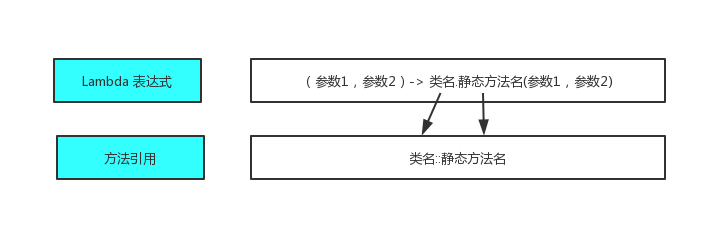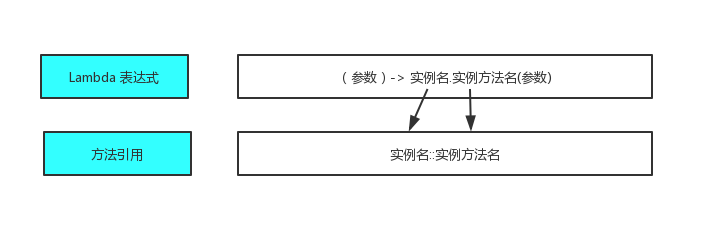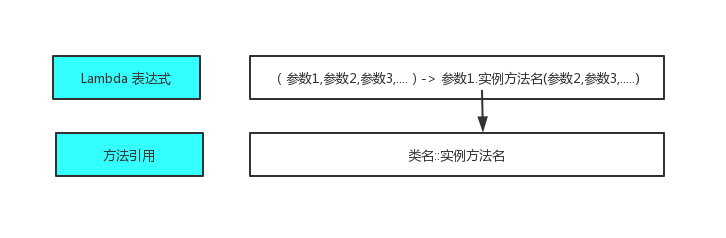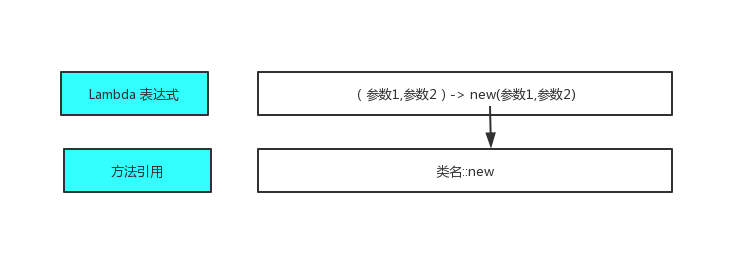# JAVA8 之 Lambda 表达式与方法引用（二） - 当年明月123

Java 的 Lambda 表达式是一种匿名函数，它是没有声明的方法，即没有访问修饰符，返回值声明和名字。

Lambda 表达式到底是什么类型那？

Lambda 表达式是一个对象。我们通过 getClass 来看一下 Lambda 表达式到底是一个什么对象。

``````public class Test8 {
public static void main(String[] args) {
Runnable r = ()-> System.out.println("Lambda");
System.out.println(r.getClass());
System.out.println(r.getClass().getInterfaces().getClass());

}
}
``````
``````class Test8\$\$Lambda\$1/1607521710
class java.lang.Class
``````

Lambda 表达式的写法

• 一个 Lambada 表达式可以有零个或者多个参数，参数的类型即可以明确声明，编译器也可以根据上下文来推断。例如 (int a) 与 (a) 效果相同。
• 所有参数需要包裹在圆括号内，参数之间用逗号隔开。空圆括号代表参数集为空。
• 当只有一个参数，且其类型可以推到时，圆括号可以省略。a->return a*a;
• Lambda 表达式的主体可以包含零条或者多条语句。如果 Lambda 表达式的主体只有一条语句，花括号{}可以省略。
``````(argument）-> {body}    //一个入参->函数体

(arg1, arg2) ->{body}   //两个入参->函数体

(Type arg1,Type arg2)->{body}

(int a,int b)->{return a+b;}   //加法操作

()->System.out.println("Hello World");  //打印 hello world

(String str)->{System.out.println(str);} //打印输入的 str；

()->100 //直接返回数字 100

()->{ return 3.1 } //直接返回 3.1
``````

Lambda 表达式的作用

``````//给调用者的方法类
public class CalUtil {

public static int cal1(int a){
return 2*a*a;
}

public static int cal2(int a){
return a > 50 && a < 60? 60:a;
}

public static int cal3(int a){
return a - 5;
}

}
``````

``````//一个 int 输入，一个 int 输出的行为抽象而成的函数式接口
@FunctionalInterface
public interface Calculate<T> {
T cal(T a);
}
``````

``````public class ClassUtils2 {
public static int compute(int a, Calculate<Integer> calculate){
//calculate 相当于一个行为，这就是我们所说的传递行为.
// 函数式接口本质上还是一个匿名内部类，一个对象
return calculate.cal(a);
}
}
``````

``````public class Test {
public static void main(String[] args) {
//输入 a，如果 a 大于 50 小于 60 则返回 60，否则返回 a
ClassUtils2.compute(50, a -> a > 50 && a < 60? 60:a);
//输入 a, 返回 a 的平方
ClassUtils2.compute(2, a->a*a);
}
}
``````

1. 需求：

将集合中的 String 转换成大写。

2. 实现：

还是使用上面那个自己定义的函数式接口。

``````@FunctionalInterface
public interface Calculate<T> {
T cal(T a);
}
``````

提供给调用者的静态方法，用于遍历 List，然后调用函数式接口的处理方法，这个方法需要调用者自己实现。

``````public static List<String> convert(List<String> inputs,Calculate<String> calculate){
List<String> res = new ArrayList<>();
for(String s:inputs){
String newString = calculate.cal(s);
}
return res;
}
``````

那么我们使用 Lambda 表达式的方式会怎么做那？

整个从小写字母到大些字母的转换过程都需要我们自己实现。

``````List<String> inputs = Arrays.asList("hello","world","java8","learning");
List<String> ls = ClassUtils2.convert(inputs,str->{
return str.toUpperCase();
});
``````

使用方法引用的方式会怎么做那？

``````List<String> inputs = Arrays.asList("hello","world","java8","learning");
List<String> ls2 = ClassUtils2.convert(inputs,String::toUpperCase);
``````

这说明 toUpperCase 方法正好满足我们自己定义的函数式接口 Calculate 的要求，一个输入，一个输出，同时它正好是实现了字符串从小写字母到大写字母的转换。所以这里就可以使用方法引用的方式 String::toUpperCase 来构建函数式接口的实例。

这里有几个需要注意的点，第一个，对于 toUpperCase 方法来说，它是没有入参的，为什么能满足上面的函数式接口那？第二个，String::toUpperCase 语法中，String 是类，toUpperCase 是实例方法，如果按照我们的理解，类调用实例方法是不对的，这个该如何理解那？

我们先看完例子介绍，下一章节给出答案。

``````   public String toUpperCase() {
}
``````

1. 需求：

将结合中的 String 字符串打印出来。

2. 实现：

由于 forEach 等方法现在还没有介绍，我们先用 for 循环的方式实现。我们先定义一个有一个输入，没有输出的函数式接口。

``````public interface Display<T>{
void display(T t);
}
``````

给调用者提供的静态方法：

``````public static void display(List<String> inputs,Display<String> d){
List<String> res = new ArrayList<>();
for(String s:inputs){
d.display(s);
}
}
``````

我们看看 Lambda 表达式的方式如何实现:

``````List<String> inputs = Arrays.asList("hello","world","java8","learning");
ClassUtils2.display(inputs,str->System.out.println(str));
``````

使用方法引用的方式：

``````ClassUtils2.display(inputs,System.out::println);
``````

我们再来看看这个 println 方法的定义，我们发现它正好满足有一个输入并且没有输出的函数式接口的约束，所以可以使用函数式接口替换 Lambda 表达式。

``````public void println(String x) {
synchronized (this) {
print(x);
newLine();
}
}
``````

• 类名::静态方法

要求静态方法的输入和输出和函数式接口一样，将 Lambda 替换为 方法引用时，必须要求这个方法客观存在。可以理解为用类调用它的静态方法。

假如我们需要根据成绩为学生排名：

我们先定义一个静态方法用来根据学生的成绩进行比较

``````public class StudentUtil {
public static int compareByScore(Student s1,Student s2){
return s1.getMark()-s2.getMark();
}
}
``````

我们使用 List 接口的 sort 方法来进行比较，这个 sort 方法接收一个函数式接口 Comparator 的实例。这个函数式接口需要两个相同的对象输入，一个 int 值输出。刚好和我们定义的静态方法吻合。

``````int compare(T o1, T o2);
``````

我们既可以使用 Lambda 表达式也可以使用方法引用的方式来进行比较：

``````public class Test2 {

public static void main(String[] args) {
Student s1 = new Student("zhang",60,"Male");
Student s2 = new Student("li",80,"Female");
Student s3 = new Student("zhao",90,"Male");
Student s4 = new Student("wang",100,"Female");

List<Student> students = Arrays.asList(s1,s2,s3,s4);

students.sort((ss1,ss2)->StudentUtil.compareByScore(ss1,ss2));

students.sort(StudentUtil::compareByScore);
}
}
``````

我们通过一个图片来让大家理清 Lambda 表达式和方法引用的关系：• 对象引用名::实例方法名

可以理解为实例对象调用实例方法。

假如我们需要返回一个字符串的第几个字符。这里我们定义一个一个输入一个输出的函数式接口。

``````@FunctionalInterface
public interface Function<T,R> {
R cal(T a);
}
``````

我们先创建一个 String s 实例，然后通过方法引用调用实例 s 的 实例方法 charAt。

``````public class Test3 {
public static void main(String[] args) {
String s = new String("hello");
Function<Integer, Character> f = s::charAt;
Character c2 = f.cal(4);

System.out.println(c2);
}
}
``````

charAt 满足一个 int 输入，一个 char 输出。

``````public char charAt(int index) {
if ((index < 0) || (index >= value.length)) {
throw new StringIndexOutOfBoundsException(index);
}
return value[index];
}
``````

我们通过一个图片来让大家理清 Lambda 表达式和方法引用的关系：• 类名::实例方法名

其实也是实例对象调用实例方法。

上面的 String::toUpperCase 就是这样一个例子，类名和实例方法的组合让大家很不解（问题1）。而且 toUpperCase 是没有入参的，但是也满足一个输入一个输出的函数式接口也是大家困惑的地方（问题2）。

`类名::实例方法名`来使用方法引用时，会将函数式接口中要求的入参中的第一个参数作为调用这个实例方法的调用者，其余参数传入实例方法中。这样上面的问题就有了答案。

问题1：归根结底，还是实例对象调用实例方法。实例对象是 Lambda 表达式的第一个参数。

问题2：虽然 toUpperCase 没有入参，但是`类名::实例方法名`的调用方式，会将调用的实例对象算作一个入参。这样就满足了一个入参，一个返回值的规则。

我们把上面的代码拿过来：

String::toUpperCase 这个方法引用中，通过 List 中的每一个 String 实例对象来调用 toUpperCase 方法。

``````List<String> inputs = Arrays.asList("hello","world","java8","learning");
List<String> ls2 = ClassUtils2.convert(inputs,String::toUpperCase);
``````

我们通过一个图片来让大家理清 Lambda 表达式和方法引用的关系：• 类名::new

实际上就是调用这个类的构造方法来生成一个对象。

我们通过 Lambda 表达式和方法引用的方式分别来返回一个对象。使用这种方式的时候，函数式接口要求的入参会全部放入到构造函数中。

我们先定义一个 Person 对象，它需要 name 和 age 两个属性来构建：

``````public class Person {

private String name;
private int age;

public Person(String name, int age) {
this.name = name;
this.age = age;
}

public String getName() {
return name;
}

public void setName(String name) {
this.name = name;
}

public int getAge() {
return age;
}

public void setAge(int age) {
this.age = age;
}
}
``````

我们在定义一个两个入参，一个返回值的函数式接口：

``````public interface BiFunction<T,R,U> {
U apply(T t,R r);
}

``````

用这个函数式接口来构建 Person：

``````public class Test4 {

BiFunction<String,Integer,Person> biFunction1 = (name,age)-> new Person(name,age);

BiFunction<String,Integer,Person> biFunction2 = Person::new;

Person p1 = biFunction1.apply("wang",18);

Person p2 = biFunction2.apply("li",20);
}

``````

我们通过一个图片来让大家理清 Lambda 表达式和方法引用的关系：0 回复

• 请尽量让自己的回复能够对别人有帮助
• 支持 Markdown 格式, **粗体**、~~删除线~~、``单行代码``
• 支持 @ 本站用户；支持表情（输入 : 提示），见 Emoji cheat sheet
• 图片支持拖拽、截图粘贴等方式上传

Java 的 Lambda 表达式是一种匿名函数，它是没有声明的方法，即没有访问修饰符，返回值声明和名字。

Lambda 表达式到底是什么类型那？

Lambda 表达式是一个对象。我们通过 getClass 来看一下 Lambda 表达式到底是一个什么对象。

``````public class Test8 {
public static void main(String[] args) {
Runnable r = ()-> System.out.println("Lambda");
System.out.println(r.getClass());
System.out.println(r.getClass().getInterfaces().getClass());

}
}
``````
``````class Test8\$\$Lambda\$1/1607521710
class java.lang.Class
``````

Lambda 表达式的写法

• 一个 Lambada 表达式可以有零个或者多个参数，参数的类型即可以明确声明，编译器也可以根据上下文来推断。例如 (int a) 与 (a) 效果相同。
• 所有参数需要包裹在圆括号内，参数之间用逗号隔开。空圆括号代表参数集为空。
• 当只有一个参数，且其类型可以推到时，圆括号可以省略。a->return a*a;
• Lambda 表达式的主体可以包含零条或者多条语句。如果 Lambda 表达式的主体只有一条语句，花括号{}可以省略。
``````(argument）-> {body}    //一个入参->函数体

(arg1, arg2) ->{body}   //两个入参->函数体

(Type arg1,Type arg2)->{body}

(int a,int b)->{return a+b;}   //加法操作

()->System.out.println("Hello World");  //打印 hello world

(String str)->{System.out.println(str);} //打印输入的 str；

()->100 //直接返回数字 100

()->{ return 3.1 } //直接返回 3.1
``````

Lambda 表达式的作用

``````//给调用者的方法类
public class CalUtil {

public static int cal1(int a){
return 2*a*a;
}

public static int cal2(int a){
return a > 50 && a < 60? 60:a;
}

public static int cal3(int a){
return a - 5;
}

}
``````

``````//一个 int 输入，一个 int 输出的行为抽象而成的函数式接口
@FunctionalInterface
public interface Calculate<T> {
T cal(T a);
}
``````

``````public class ClassUtils2 {
public static int compute(int a, Calculate<Integer> calculate){
//calculate 相当于一个行为，这就是我们所说的传递行为.
// 函数式接口本质上还是一个匿名内部类，一个对象
return calculate.cal(a);
}
}
``````

``````public class Test {
public static void main(String[] args) {
//输入 a，如果 a 大于 50 小于 60 则返回 60，否则返回 a
ClassUtils2.compute(50, a -> a > 50 && a < 60? 60:a);
//输入 a, 返回 a 的平方
ClassUtils2.compute(2, a->a*a);
}
}
``````

1. 需求：

将集合中的 String 转换成大写。

2. 实现：

还是使用上面那个自己定义的函数式接口。

``````@FunctionalInterface
public interface Calculate<T> {
T cal(T a);
}
``````

提供给调用者的静态方法，用于遍历 List，然后调用函数式接口的处理方法，这个方法需要调用者自己实现。

``````public static List<String> convert(List<String> inputs,Calculate<String> calculate){
List<String> res = new ArrayList<>();
for(String s:inputs){
String newString = calculate.cal(s);
}
return res;
}
``````

那么我们使用 Lambda 表达式的方式会怎么做那？

整个从小写字母到大些字母的转换过程都需要我们自己实现。

``````List<String> inputs = Arrays.asList("hello","world","java8","learning");
List<String> ls = ClassUtils2.convert(inputs,str->{
return str.toUpperCase();
});
``````

使用方法引用的方式会怎么做那？

``````List<String> inputs = Arrays.asList("hello","world","java8","learning");
List<String> ls2 = ClassUtils2.convert(inputs,String::toUpperCase);
``````

这说明 toUpperCase 方法正好满足我们自己定义的函数式接口 Calculate 的要求，一个输入，一个输出，同时它正好是实现了字符串从小写字母到大写字母的转换。所以这里就可以使用方法引用的方式 String::toUpperCase 来构建函数式接口的实例。

这里有几个需要注意的点，第一个，对于 toUpperCase 方法来说，它是没有入参的，为什么能满足上面的函数式接口那？第二个，String::toUpperCase 语法中，String 是类，toUpperCase 是实例方法，如果按照我们的理解，类调用实例方法是不对的，这个该如何理解那？

我们先看完例子介绍，下一章节给出答案。

``````   public String toUpperCase() {
}
``````

1. 需求：

将结合中的 String 字符串打印出来。

2. 实现：

由于 forEach 等方法现在还没有介绍，我们先用 for 循环的方式实现。我们先定义一个有一个输入，没有输出的函数式接口。

``````public interface Display<T>{
void display(T t);
}
``````

给调用者提供的静态方法：

``````public static void display(List<String> inputs,Display<String> d){
List<String> res = new ArrayList<>();
for(String s:inputs){
d.display(s);
}
}
``````

我们看看 Lambda 表达式的方式如何实现:

``````List<String> inputs = Arrays.asList("hello","world","java8","learning");
ClassUtils2.display(inputs,str->System.out.println(str));
``````

使用方法引用的方式：

``````ClassUtils2.display(inputs,System.out::println);
``````

我们再来看看这个 println 方法的定义，我们发现它正好满足有一个输入并且没有输出的函数式接口的约束，所以可以使用函数式接口替换 Lambda 表达式。

``````public void println(String x) {
synchronized (this) {
print(x);
newLine();
}
}
``````

• 类名::静态方法

要求静态方法的输入和输出和函数式接口一样，将 Lambda 替换为 方法引用时，必须要求这个方法客观存在。可以理解为用类调用它的静态方法。

假如我们需要根据成绩为学生排名：

我们先定义一个静态方法用来根据学生的成绩进行比较

``````public class StudentUtil {
public static int compareByScore(Student s1,Student s2){
return s1.getMark()-s2.getMark();
}
}
``````

我们使用 List 接口的 sort 方法来进行比较，这个 sort 方法接收一个函数式接口 Comparator 的实例。这个函数式接口需要两个相同的对象输入，一个 int 值输出。刚好和我们定义的静态方法吻合。

``````int compare(T o1, T o2);
``````

我们既可以使用 Lambda 表达式也可以使用方法引用的方式来进行比较：

``````public class Test2 {

public static void main(String[] args) {
Student s1 = new Student("zhang",60,"Male");
Student s2 = new Student("li",80,"Female");
Student s3 = new Student("zhao",90,"Male");
Student s4 = new Student("wang",100,"Female");

List<Student> students = Arrays.asList(s1,s2,s3,s4);

students.sort((ss1,ss2)->StudentUtil.compareByScore(ss1,ss2));

students.sort(StudentUtil::compareByScore);
}
}
``````

我们通过一个图片来让大家理清 Lambda 表达式和方法引用的关系：• 对象引用名::实例方法名

可以理解为实例对象调用实例方法。

假如我们需要返回一个字符串的第几个字符。这里我们定义一个一个输入一个输出的函数式接口。

``````@FunctionalInterface
public interface Function<T,R> {
R cal(T a);
}
``````

我们先创建一个 String s 实例，然后通过方法引用调用实例 s 的 实例方法 charAt。

``````public class Test3 {
public static void main(String[] args) {
String s = new String("hello");
Function<Integer, Character> f = s::charAt;
Character c2 = f.cal(4);

System.out.println(c2);
}
}
``````

charAt 满足一个 int 输入，一个 char 输出。

``````public char charAt(int index) {
if ((index < 0) || (index >= value.length)) {
throw new StringIndexOutOfBoundsException(index);
}
return value[index];
}
``````

我们通过一个图片来让大家理清 Lambda 表达式和方法引用的关系：• 类名::实例方法名

其实也是实例对象调用实例方法。

上面的 String::toUpperCase 就是这样一个例子，类名和实例方法的组合让大家很不解（问题1）。而且 toUpperCase 是没有入参的，但是也满足一个输入一个输出的函数式接口也是大家困惑的地方（问题2）。

`类名::实例方法名`来使用方法引用时，会将函数式接口中要求的入参中的第一个参数作为调用这个实例方法的调用者，其余参数传入实例方法中。这样上面的问题就有了答案。

问题1：归根结底，还是实例对象调用实例方法。实例对象是 Lambda 表达式的第一个参数。

问题2：虽然 toUpperCase 没有入参，但是`类名::实例方法名`的调用方式，会将调用的实例对象算作一个入参。这样就满足了一个入参，一个返回值的规则。

我们把上面的代码拿过来：

String::toUpperCase 这个方法引用中，通过 List 中的每一个 String 实例对象来调用 toUpperCase 方法。

``````List<String> inputs = Arrays.asList("hello","world","java8","learning");
List<String> ls2 = ClassUtils2.convert(inputs,String::toUpperCase);
``````

我们通过一个图片来让大家理清 Lambda 表达式和方法引用的关系：• 类名::new

实际上就是调用这个类的构造方法来生成一个对象。

我们通过 Lambda 表达式和方法引用的方式分别来返回一个对象。使用这种方式的时候，函数式接口要求的入参会全部放入到构造函数中。

我们先定义一个 Person 对象，它需要 name 和 age 两个属性来构建：

``````public class Person {

private String name;
private int age;

public Person(String name, int age) {
this.name = name;
this.age = age;
}

public String getName() {
return name;
}

public void setName(String name) {
this.name = name;
}

public int getAge() {
return age;
}

public void setAge(int age) {
this.age = age;
}
}
``````

我们在定义一个两个入参，一个返回值的函数式接口：

``````public interface BiFunction<T,R,U> {
U apply(T t,R r);
}

``````

用这个函数式接口来构建 Person：

``````public class Test4 {

BiFunction<String,Integer,Person> biFunction1 = (name,age)-> new Person(name,age);

BiFunction<String,Integer,Person> biFunction2 = Person::new;

Person p1 = biFunction1.apply("wang",18);

Person p2 = biFunction2.apply("li",20);
}

``````

我们通过一个图片来让大家理清 Lambda 表达式和方法引用的关系：